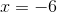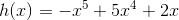### Test: CLEP Calculus

 1 Is the following function increasing or decreasing at the point?h(x) is increasing at, because the second derivative is positive.

h(x) is increasing at, because the first derivative is positive.

h(x) is decreasing at, because the second derivative is negative.

h(x) is decreasing at, because the first derivative is negative.

1/2 questions

0%

## Access results and powerful study features!

Take 15 seconds to create an account.